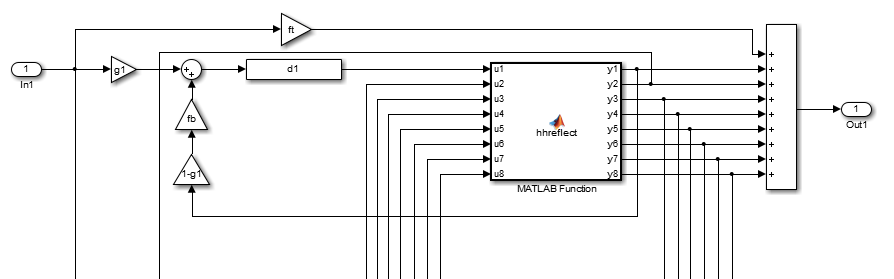# Generate Accelerated MEX Code for Reverberation Using MATLAB® Classes

This example shows how to accelerate the execution of a MATLAB algorithm that uses MATLAB classes. The classes create a reverberation effect, that is, the "echo" you hear in a large empty room.

### Implementing a Simple Reverberation Effect

There are many ways to implement a reverberation effect with different characteristics. In terms of audio quality, this is not an advanced effect, but shows the capabilities of using MATLAB classes with MATLAB Coder™.

This reverberation effect is implemented based on the following block diagram:The diagram shows only the first delay line. Imagine another seven delay lines being repeated in the diagram but each delay line has an individual delay and associated feedback gain block. The Householder reflection (i.e. `hhreflect` function) is essentially mixing/permuting the signals without changing the energy of the total signal. Therefore, we are essentially duplicating the incoming signal and feeding it back with small time displacements. The result is a reverberation effect.

### Files Used

• `reverb_test.m`: Main file testing the reverberation effect

• `do_reverb.m`: Function abstraction of the Reverb class

• `Reverb.m`: Effect implementation implemented as a MATLAB class

• `Delay.m`: Delay effect for `Reverb.m` implemented as a MATLAB class

• `hhreflect.m`: Householder reflection for `Reverb.m`

• `get_prime.m`: Function to compute prime numbers (for `Reverb.m`)

• `speech_dft.mat`: Test sample file

### Generate a MEX Function

`codegen do_reverb`
```Code generation successful. ```

### Run the MEX Function

This processes the sample file (`speech_dft.mat`), applies the reverberation effect, and outputs the result to the computer's audio output.

`reverb_test;`
```Running time = 16 milliseconds ```

### Generate a Faster MEX Function

Disable the integrity checks (e.g. out of bound checks for matrices) to obtain a faster but potentially unsafe MEX function.

```cfg = coder.config; cfg.IntegrityChecks = false; codegen -config cfg do_reverb```
```Code generation successful. ```

### Retry the MEX Function

`reverb_test;`
```Running time = 7 milliseconds ```

## SupportGet trial now